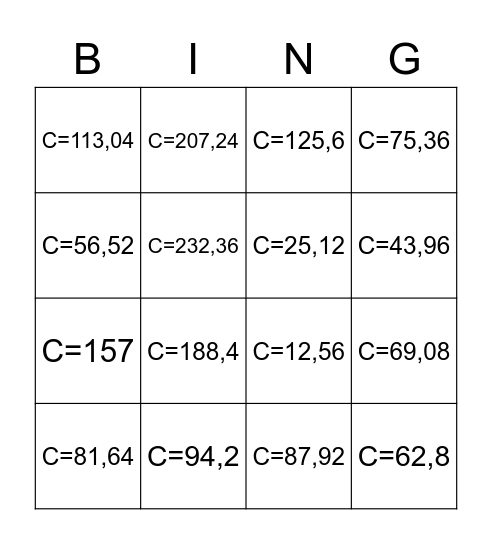# Bingo da CircunferênciaThis bingo card has 41 words: Free!, C=12,56, C=18,84, C=25,12, C=31,4, C=37,68, C=43,96, C=50,24, C=56,52, C=62,8, C=69,08, C=75,36, C=81,64, C=87,92, C=94,2, C=100,48, C=106,76, C=113,04, C=119,32, C=125,6, C=131,88, C=144,44, C=150,72, C=157, C=163,28, C=169,56, C=175,84, C=182,12, C=188,4, C=194,68, C=200,96, C=207,24, C=213,52, C=219,8, C=226,08, C=232,36, C=238,64, C=244,92, C=251,2, C=257,48 and C=263,76.

## Play Online